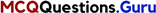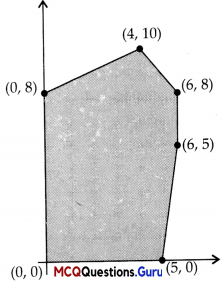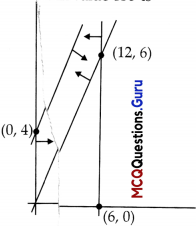# HSSlive: Plus One & Plus Two Notes & Solutions for Kerala State Board

## MCQ Questions for Class 12 Maths Chapter 12 Linear Programming With Answers PDFMCQ Questions for Class 12 Maths Chapter 12 Linear Programming With Answers PDF

MCQ Questions for Class 12 Maths Chapter 12 Linear Programming With Answers PDF: Multiple Choice Questions (MCQs) are a popular method of testing a student's understanding of a particular subject. They are widely used in school and college exams as they are a quick and efficient way to assess a student's knowledge.

An MCQ Questions for Class 12 Maths Chapter 12 Linear Programming consists of a question or statement, followed by several answer options, of which the student must choose the correct one. The answer options are usually presented as a list of possible choices, such as A, B, C or D.

## MCQ Questions for Class 12 Maths Chapter 12 Linear Programming With Answers PDF

Linear Programming Class 12 MCQ Chapter 12 Question 1.
The corner points of the feasible region determined by the system of linear constraints are (0,0), (0,40), (20,40), (60,20), (60,0). The objective function is Z = 4x + 3y.
Compare the quantity in Column A and Column B

 Column A Column B Maximum of Z 325

(A) The quantity in column A is greater.
(B) The quantity in column B is greater.
(C) The two quantities are equal.
(D) The relationship cannot be determined on the basis of the information supplied.
(B) The quantity in column B is greater.

Explanation:

 Corner points Corresponding value of Z = 4x + 3y (0, 0) 0 (0, 40) 120 (20, 40) 200 (60, 20) 300 ←Maximum (60, 0) 240

Hence, maximum value of Z = 300 < 325 So, the quantity in column B is greater.MCQ Questions On Linear Programming Class 12 Question 2.
The feasible solution for a LPP is si iown in given figure. Let Z = 3x – 4y be the objective function Minimum of Z occurs at(A) (0,0)
(B) (0,8)
(C) (5,0)
(D) (4,10)
(B) (0,8)

Explanation:

 Corner points Corresponding value of Z = 3x – 4y (0,0) 0 (5,0) 15 ←Maximum (6,5) -2 (6,8) -14 (4, 10) -28 (0, 8) -32 ←Minimum

Hence, the minimum of Z occurs at (0, 8) and its minimum value is (-32).

Linear Programming MCQ Chapter 12 Class 12 Question 3.
The feasible solution for a LPP is si iown in given figure. Let Z = 3x – 4y be the obje .ctive function Minimum of Z, maximum of Z occurs at
(A) (5,0)
(B) (6,5)
(C) (6, 8)
(D) (4,10)
(A) (5,0)

Explanation:
Maximum of Z occurs at (5, 0).

Linear Programming MCQ With Answers Pdf Question 4.
The feasible solution for a LPP is si iown in given figure. Let Z = 3x – 4y be the obje .ctive function Minimum of Z , (Maximum va lue of Z + Minimum value of Z) is equal to ……………
(A ) 13
(B) 1
(C) -13
(D) -17
(D) -17

Explanation:
Maximum value of Z + Minimum value of Z = 15 – 32 = -17

MCQ Of Linear Programming Class 12 Question 5.
The t easible region for an LPP is shown in the given Figure. Let F = 3x – 4y be the objective function. Maximum value of F is …………..(A) 0
(B) 8
(C) 12
(D) -18
(C) 12

Explanation:
The feasible region as shown in the figure, has objective function F = 3x – 4y.

 Corner points Corresponding value of F = 3x – 4y (0,0) 0 (12,6) 12 ←Maximum (0,4) -16 ←Maximum

Hence, the maximum, value of F is 12.

## Benefits of MCQ Questions for Class 12 Maths Chapter 12 Linear Programming

One of the benefits of using MCQ Questions for Class 12 Maths Chapter 12 Linear Programming is that they are easy to grade. The correct answer is already provided, so the teacher or exam proctor simply needs to check that the student has chosen the correct option. This makes MCQ Questions for Class 12 Maths Chapter 12 Linear Programming more efficient than open-ended questions, which require more time and effort to grade.

Another benefit of MCQ Questions for Class 12 Maths Chapter 12 Linear Programming is that they can test a wide range of knowledge, including factual information, concepts, and problem-solving skills. They can also be used to test a student's ability to apply knowledge to new situations, rather than just recall information.

## Conclusion of MCQ Questions for Class 12 Maths Chapter 12 Linear Programming With Answers PDF

In conclusion, MCQ Questions for Class 12 Maths Chapter 12 Linear Programming are a popular method of testing a student's understanding of a particular subject. They are widely used in school and college exams as they are a quick and efficient way to assess a student's knowledge. But to minimize the drawbacks, it is important to write good MCQs and use a variety of question types. They can test a wide range of knowledge, including factual information, concepts, and problem-solving skills, but they should not be the only method of assessment, it's important to use different types of questions to evaluate a student's understanding of a subject.

## FAQs About MCQ Questions for Class 12 Maths Chapter 12 Linear Programming

#### Q: What are Multiple Choice Questions (MCQs)??

A: MCQs are a popular method of testing a student's understanding of a particular subject. They consist of a question or statement, followed by several answer options, of which the student must choose the correct one.

#### Q: Why are MCQ Questions for Class 12 Maths Chapter 12 Linear Programming used in exams?

A: MCQs are widely used in school and college exams as they are a quick and efficient way to assess a student's knowledge. They are easy to grade as the correct answer is already provided, making it more efficient than open-ended questions.

#### Q: What are the different types of MCQs?

A: The different types of MCQs are true/false, multiple choice, matching, and completion.

## MCQ Question for Class 12 Maths All Chapters

• MCQ Questions for Class 12 Maths Chapter 1 Relation and Function With Answers PDF
• MCQ Questions for Class 12 Maths Chapter 2 Inverse Trigonometric Functions With Answers PDF
• MCQ Questions for Class 12 Maths Chapter 3 Matrices With Answers PDF
• MCQ Questions for Class 12 Maths Chapter 4 Determinants With Answers PDF
• MCQ Questions for Class 12 Maths Chapter 5 Continuity and Differentiability With Answers PDF
• MCQ Questions for Class 12 Maths Chapter 6 Application of Derivatives With Answers PDF
• MCQ Questions for Class 12 Maths Chapter 7 Integrals With Answers PDF
• MCQ Questions for Class 12 Maths Chapter 8 Application of Integrals With Answers PDF
• MCQ Questions for Class 12 Maths Chapter 9 Differential Equations With Answers PDF
• MCQ Questions for Class 12 Maths Chapter 10 Vector Algebra With Answers PDF
• MCQ Questions for Class 12 Maths Chapter 11 Three Dimensional Geometry With Answers PDF
• MCQ Questions for Class 12 Maths Chapter 12 Linear Programming With Answers PDF
• MCQ Questions for Class 12 Maths Chapter 13 Probability With Answers PDF
• Share: